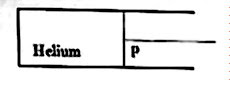## Pages

`“Life is like riding a bicycle.  To keep your balance you must keep moving.”–Albert Einstein`

## Saturday, March 15, 2008

### AP Physics B – Practice Questions (MCQ) on Kinetic Theory

As promised in the post dated 13th March 2007, I give below some typical multiple choice questions on kinetic theory for practice. For the time being I leave these questions here without solution.

(1) If a diatomic gas molecule has an additional vibrational mode which contributes to both kinetic and potential energies, the sum of which is equal to kT where k is Boltzmann’s constant and T is the absolute temperature, what is the ratio of specific heats of the gas?

(a) 3/2

(b) 4/3

(c) 5/3

(d) 7/5

(e) 9/7

(2)
Four moles of helium (a mono atomic gas) is contained in a barrel provided with a light, frictionless piston (fig.). The quantity of heat to be supplied to the gas for raising its temperature by 5 K is (Universal gas constant, R = 8.31 J mol–1 K–1)(a) 415.5 J

(b) 249.3 J

(c) 136.5 J

(d) 166.2 J

(e) 581.7 J

(3)
The intermolecular attraction is an important reason why real gases behave differently compared to ideal gas. But real gases too can behave like ideal gas at

(a) low temperature and low pressure

(b) low temperature and high pressure

(c) high temperature and high pressure

(d) high temperature and low pressure

(e) absolute zero (0 K) temperature

(4)
A fixed mass of an ideal gas at pressure P is contained in a closed vessel of volume V. It is heated so that the root mean square velocity of the gas molecules is doubled. The thermal expansion of the vessel is negligible. Then the increase in pressure (P) of the gas is

(a) P

(b) 2P

(c) 3P

(d) 4P

(e) √2 P

(5)
A cubical vessel of side contains N molecules of an ideal gas. If the mass of a molecule is m and the root mean square velocity is c, the pressure of the gas is

(a) mNc2 /3 3

(b) mNc2 /3

(c) mc2 /33

(d) 3mNc2 /3

(e) (1/3)mNc2

(6)
Four moles of oxygen (diatomic) is mixed with eight moles of neon (mono atomic). If they are treated as ideal gases, the effective molar specific heat at constant volume of the mixture is (R = universal gas constant)

(a) (5/3) R

(b) (7/5) R

(c) (9/5) R

(d) (11/6) R

(e) (13/8) R

(7) The temperature at which the root mean square velocity of hydrogen gas molecules is twice that at 10º C is

(a) 20º C

(b) 40º C

(c) 819º C

(d) 859º C

(e) 1132º C

(8) Equal number of oxygen molecules (molar mass m1) and helium molecules (molar mass m2) are kept in two identical vessels. If they are at the same temperature, their pressures will be in the ratio

(a) m1/m2

(b) m2/m1

(c) √(m1/m2)

(d) √(m2/m1)

(e) 1

Try to answer these questions. The essential points required for solving these questions were discussed in the post dated 13th March 2008, which you can access by clicking on the label ‘kinetic theory’ below this post.

I’ll be back with the solution in a day or two.